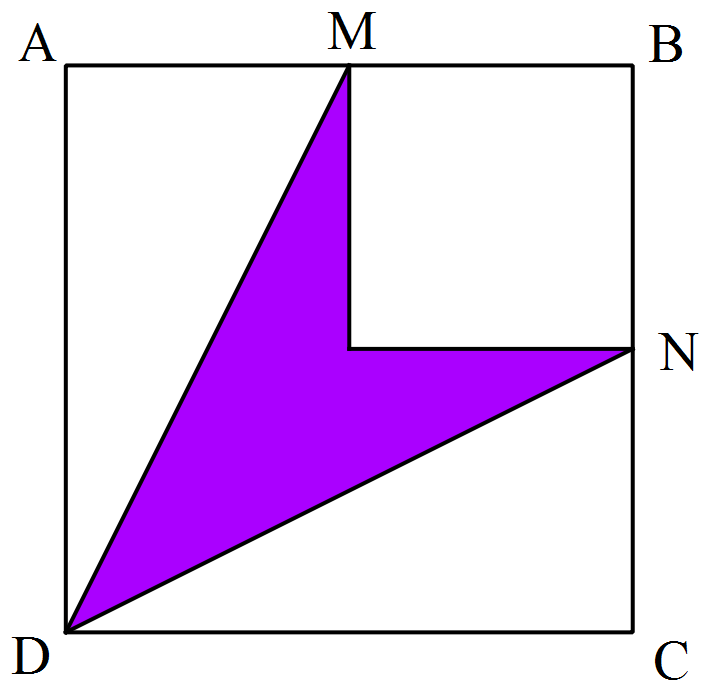# Purple Kite

Geometry Level 1$ABCD$ is a square and $M$ and $N$ are the midpoints of the side $AB$ and $BC$ respectively. What fraction of $ABCD$ is colored purple?

×